# FunctionalChaosAlgorithm¶

class FunctionalChaosAlgorithm(*args)

Functional chaos algorithm.

Available constructors:

FunctionalChaosAlgorithm(inputSample, outputSample)

FunctionalChaosAlgorithm(inputSample, outputSample, distribution)

FunctionalChaosAlgorithm(inputSample, weights, outputSample, distribution, adaptiveStrategy, projectionStrategy)

Parameters:
inputSample, outputSample2-d sequence of float

Sample of the input - output random vectors

distributionDistribution

Distribution of the random vector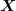adaptiveStrategyAdaptiveStrategy

Strategy of selection of the different terms of the multivariate basis.

projectionStrategyProjectionStrategy

Strategy of evaluation of the coefficients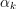weightssequence of float

Weights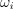associated to the data base

Default values are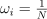where N=inputSample.getSize()

Notes

Consider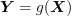with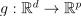,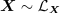and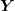with finite variance: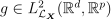.

When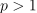, the functional chaos algorithm is used on each marginal of, using the same multivariate orthonormal basis for all the marginals. Thus, the algorithm is detailed here for a scalar output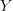and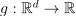.

Let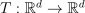be an isoprobabilistic transformation such that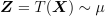. We note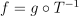, then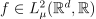.

Let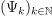be an orthonormal multivariate basis of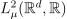.

Then the functional chaos decomposition of f writes: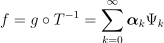which can be truncated to the finite set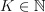: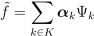The approximation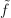can be used to build an efficient random generator ofbased on the random vector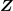. It writes: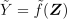For more details, see FunctionalChaosRandomVector.

The functional chaos decomposition can be used to build a meta model of g, which writes: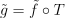If the basishas been obtained by tensorisation of univariate orthonormal basis, then the distribution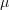writes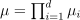. In that case only, the Sobol indices can easily be deduced from the coefficients.

We detail here all the steps required in order to create a functional chaos algorithm.

Step 1 - Construction of the multivariate orthonormal basis: the multivariate orthonornal basis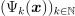is built as the tensor product of orthonormal univariate families.

The univariate bases may be:

• polynomials: the associated distribution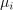is continuous or discrete. Note that it is possible to build the polynomial family orthonormal to any univariate distributionunder some conditions. For more details, see StandardDistributionPolynomialFactory;

• Haar wavelets: they enable to approximate functions with discontinuities. For more details, see HaarWaveletFactory,;

• Fourier series: for more details, see FourierSeriesFactory.

Furthermore, the numerotation of the multivariate orthonormal basis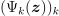is given by an enumerate function which defines a regular way to generate the collection of degres used for the univariate polynomials : an enumerate function represents a bijection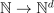. See LinearEnumerateFunction or HyperbolicAnisotropicEnumerateFunction for more details.

Step 2 - Truncation strategy of the multivariate orthonormal basis: a strategy must be chosen for the selection of the different terms of the multivariate basis. The selected terms are gathered in the subset K.

For more details on the possible strategies, see FixedStrategy and CleaningStrategy.

Step 3 - Evaluation strategy of the coefficients: a strategy must be chosen for the estimation of te coefficients. The vector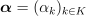is equivalently defined by:

(1)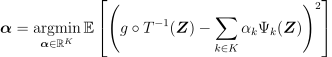or

(2)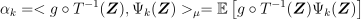where the mean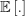is evaluated with respect to the measure.

Relation (1) means that the coefficients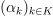minimize the quadratic error between the model and the polynomial approximation. For more details, see LeastSquaresStrategy.

Relation (2) means thatis the scalar product of the model with the k-th element of the orthonormal basis. For more details, see IntegrationStrategy.

Examples

Create the model:

>>> import openturns as ot
>>> ot.RandomGenerator.SetSeed(0)
>>> inputDim = 1
>>> model = ot.SymbolicFunction(['x'], ['x*sin(x)'])
>>> distribution = ot.ComposedDistribution([ot.Uniform()]*inputDim)


Build the multivariate orthonormal basis:

>>> polyColl = [0.0]*inputDim
>>> for i in range(distribution.getDimension()):
...     polyColl[i] = ot.StandardDistributionPolynomialFactory(distribution.getMarginal(i))
>>> enumerateFunction = ot.LinearEnumerateFunction(inputDim)
>>> productBasis = ot.OrthogonalProductPolynomialFactory(polyColl, enumerateFunction)


Define the strategy to truncate the multivariate orthonormal basis: We choose all the polynomials of degree <= 4

>>> degree = 4
>>> indexMax = enumerateFunction.getStrataCumulatedCardinal(degree)
>>> print(indexMax)
5


We keep all the polynomials of degree <= 4 (which corresponds to the 5 first ones):

>>> adaptiveStrategy = ot.FixedStrategy(productBasis, indexMax)


Define the evaluation strategy of the coefficients:

>>> samplingSize = 50
>>> experiment = ot.MonteCarloExperiment(distribution, samplingSize)
>>> X = experiment.generate()
>>> Y = model(X)
>>> projectionStrategy = ot.LeastSquaresStrategy()


Create the chaos algorithm:

>>> algo = ot.FunctionalChaosAlgorithm(X, Y, distribution, adaptiveStrategy,
...                                    projectionStrategy)
>>> algo.run()


Get the result:

>>> functionalChaosResult = algo.getResult()
>>> metamodel = functionalChaosResult.getMetaModel()


Test it:

>>> X = [0.5]
>>> print(model(X))
[0.239713]
>>> print(metamodel(X))
[0.239514]


Methods

 BuildDistribution(inputSample) Recover the distribution, with metamodel performance in mind. Get the adaptive strategy. Accessor to the object's name. Accessor to the joint probability density function of the physical input vector. Accessor to the object's id. Accessor to the input sample. Get the maximum residual. Accessor to the object's name. Accessor to the output sample. Get the projection strategy. Get the results of the metamodel computation. Accessor to the object's shadowed id. Accessor to the object's visibility state. Test if the object is named. Test if the object has a distinguishable name. Compute the metamodel. setDistribution(distribution) Accessor to the joint probability density function of the physical input vector. setMaximumResidual(residual) Set the maximum residual. setName(name) Accessor to the object's name. setProjectionStrategy(projectionStrategy) Set the projection strategy. Accessor to the object's shadowed id. setVisibility(visible) Accessor to the object's visibility state.
__init__(*args)
static BuildDistribution(inputSample)

Recover the distribution, with metamodel performance in mind.

For each marginal, find the best 1-d continuous parametric model else fallback to the use of a nonparametric one.

The selection is done as follow:

• We start with a list of all parametric models (all factories)

• For each model, we estimate its parameters if feasible.

• We check then if model is valid, ie if its Kolmogorov score exceeds a threshold fixed in the MetaModelAlgorithm-PValueThreshold ResourceMap key. Default value is 5%

• We sort all valid models and return the one with the optimal criterion.

For the last step, the criterion might be BIC, AIC or AICC. The specification of the criterion is done through the MetaModelAlgorithm-ModelSelectionCriterion ResourceMap key. Default value is fixed to BIC. Note that if there is no valid candidate, we estimate a non-parametric model (KernelSmoothing or Histogram). The MetaModelAlgorithm-NonParametricModel ResourceMap key allows selecting the preferred one. Default value is Histogram

One each marginal is estimated, we use the Spearman independence test on each component pair to decide whether an independent copula. In case of non independence, we rely on a NormalCopula.

Parameters:
sampleSample

Input sample.

Returns:
distributionDistribution

Input distribution.

Returns:
adaptiveStrategyAdaptiveStrategy

Strategy of selection of the different terms of the multivariate basis.

getClassName()

Accessor to the object’s name.

Returns:
class_namestr

The object class name (object.__class__.__name__).

getDistribution()

Accessor to the joint probability density function of the physical input vector.

Returns:
distributionDistribution

Joint probability density function of the physical input vector.

getId()

Accessor to the object’s id.

Returns:
idint

Internal unique identifier.

getInputSample()

Accessor to the input sample.

Returns:
inputSampleSample

Input sample of a model evaluated apart.

getMaximumResidual()

Get the maximum residual.

Returns:
residualfloat

Residual value needed in the projection strategy.

Default value is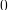.

getName()

Accessor to the object’s name.

Returns:
namestr

The name of the object.

getOutputSample()

Accessor to the output sample.

Returns:
outputSampleSample

Output sample of a model evaluated apart.

getProjectionStrategy()

Get the projection strategy.

Returns:
strategyProjectionStrategy

Projection strategy.

Notes

The projection strategy selects the different terms of the multivariate basis to define the subset K.

getResult()

Get the results of the metamodel computation.

Returns:
resultFunctionalChaosResult

Result structure, created by the method run().

Accessor to the object’s shadowed id.

Returns:
idint

Internal unique identifier.

getVisibility()

Accessor to the object’s visibility state.

Returns:
visiblebool

Visibility flag.

hasName()

Test if the object is named.

Returns:
hasNamebool

True if the name is not empty.

hasVisibleName()

Test if the object has a distinguishable name.

Returns:
hasVisibleNamebool

True if the name is not empty and not the default one.

run()

Compute the metamodel.

Notes

Evaluates the metamodel and stores all the results in a result structure.

setDistribution(distribution)

Accessor to the joint probability density function of the physical input vector.

Parameters:
distributionDistribution

Joint probability density function of the physical input vector.

setMaximumResidual(residual)

Set the maximum residual.

Parameters:
residualfloat

Residual value needed in the projection strategy.

Default value is.

setName(name)

Accessor to the object’s name.

Parameters:
namestr

The name of the object.

setProjectionStrategy(projectionStrategy)

Set the projection strategy.

Parameters:
strategyProjectionStrategy

Strategy to estimate the coefficients.

Accessor to the object’s shadowed id.

Parameters:
idint

Internal unique identifier.

setVisibility(visible)

Accessor to the object’s visibility state.

Parameters:
visiblebool

Visibility flag.

## Examples using the class¶Mixture of experts

Mixture of expertsFit a distribution from an input sample

Fit a distribution from an input samplePolynomial chaos exploitation

Polynomial chaos exploitationPolynomial chaos over database

Polynomial chaos over databaseCompute grouped indices for the Ishigami function

Compute grouped indices for the Ishigami functionValidate a polynomial chaos

Validate a polynomial chaosCreate a polynomial chaos metamodel by integration on the cantilever beam

Create a polynomial chaos metamodel by integration on the cantilever beamCreate a polynomial chaos metamodel

Create a polynomial chaos metamodelCreate a polynomial chaos for the Ishigami function: a quick start guide to polynomial chaos

Create a polynomial chaos for the Ishigami function: a quick start guide to polynomial chaosChaos cross-validation

Chaos cross-validationPolynomial chaos is sensitive to the degree

Polynomial chaos is sensitive to the degreeCreate a sparse chaos by integration

Create a sparse chaos by integrationCompute Sobol’ indices confidence intervals

Compute Sobol' indices confidence intervalsViscous free fall: metamodel of a field function

Viscous free fall: metamodel of a field functionMetamodel of a field function

Metamodel of a field functionSobol’ sensitivity indices from chaos

Sobol' sensitivity indices from chaosUse the ANCOVA indices

Use the ANCOVA indicesExample of sensitivity analyses on the wing weight model

Example of sensitivity analyses on the wing weight model